Important Questions: Data Handling

# Important Questions: Data Handling

Test Description

## 20 Questions MCQ Test Online MCQ Tests for Class 7 | Important Questions: Data Handling

Important Questions: Data Handling for Class 7 2022 is part of Online MCQ Tests for Class 7 preparation. The Important Questions: Data Handling questions and answers have been prepared according to the Class 7 exam syllabus.The Important Questions: Data Handling MCQs are made for Class 7 2022 Exam. Find important definitions, questions, notes, meanings, examples, exercises, MCQs and online tests for Important Questions: Data Handling below.
Solutions of Important Questions: Data Handling questions in English are available as part of our Online MCQ Tests for Class 7 for Class 7 & Important Questions: Data Handling solutions in Hindi for Online MCQ Tests for Class 7 course. Download more important topics, notes, lectures and mock test series for Class 7 Exam by signing up for free. Attempt Important Questions: Data Handling | 20 questions in 20 minutes | Mock test for Class 7 preparation | Free important questions MCQ to study Online MCQ Tests for Class 7 for Class 7 Exam | Download free PDF with solutions
 1 Crore+ students have signed up on EduRev. Have you?
Important Questions: Data Handling - Question 1

### What is the arithmetic mean of 1, 2,...., 9 and 10?

Detailed Solution for Important Questions: Data Handling - Question 1

By definition, A.M.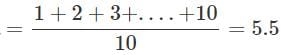By shortcut, A.M.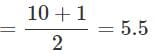Important Questions: Data Handling - Question 2

### In a data, 10 numbers are arranged in increasing order. If the 7th entry is increased by 4, by how much does the median increase?

Detailed Solution for Important Questions: Data Handling - Question 2

For 10 observations, the median would be the average of the 5th and 6th observations. Since they are unaffected by increase in 7th entry, the median will be unchanged.

Important Questions: Data Handling - Question 3

### What is the mean of x, x+3, x+6, x+9 and x+12?

Detailed Solution for Important Questions: Data Handling - Question 3

By definition, Average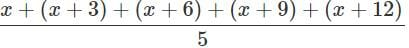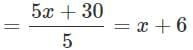Important Questions: Data Handling - Question 4

The daily sales of kerosene (in litres) in a ration shop for six days is given in the box.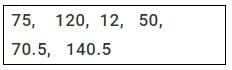What is the average daily sale?

Detailed Solution for Important Questions: Data Handling - Question 4

By definition of average, the average daily sale =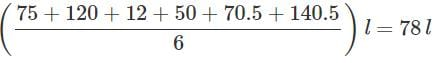However, without calculating we can say that the answer is D since the average lies between the maximum and the minimum.

Important Questions: Data Handling - Question 5

The mean of five numbers is 27. If one of the numbers is excluded, the mean gets reduced by 2. What is the excluded number?

Detailed Solution for Important Questions: Data Handling - Question 5

Let the sum of four numbers be y and the excluded number be x.
Then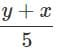=27  and , y/4 = 25 or x+y = 135 and y = 100 ⇒ x = 35

Important Questions: Data Handling - Question 6

What is the median of the data 46,64,87, 41,58,77,35,90,55,33,92?

Detailed Solution for Important Questions: Data Handling - Question 6

Arranging the given data in ascending order, we have, 33, 35, 41, 46, 55, 58, 64, 77, 87, 90 and 92. The sixth entry is 58.
∴ Median is 58.

Important Questions: Data Handling - Question 7

Which of the following is true about mean?

Important Questions: Data Handling - Question 8

If each entry of a data is increased by 5, how does the mean change?

Detailed Solution for Important Questions: Data Handling - Question 8

Let new mean be M , no. of observations be n and old sum is x
So if every entry is increased by 5 sum of observations is increased by 5n
M=(s+5n)/n
=s/n + 5n/n
=s/n +5
So the new mean is increased by 5

Important Questions: Data Handling - Question 9

The arithmetic mean of five given numbers is 85. What is their sum?

Detailed Solution for Important Questions: Data Handling - Question 9

arithmetic mean = sum of observation / total no of observation

therefore;

sum of observation = arithmetic mean x total no of observation

sum of observation = 85 X 5=425

Important Questions: Data Handling - Question 10

The average weight of a sample of 10 apples is 52 g. Later it was found that the weighing machine had shown the weight of each apple 10 g less. What is the correct average weight of an apple?

Detailed Solution for Important Questions: Data Handling - Question 10

Since every apple has now 10g more, the average will rise by 10g, So accurate average= 52+10=62g

Important Questions: Data Handling - Question 11

The mean of 6, y, 7, x and 14 is 8.Which of the following is true?

Detailed Solution for Important Questions: Data Handling - Question 11

Mean=(6+y+7+x+14)/5
8=(27+y+x)/5
40=27+y+x
y+x=40-27=13

Important Questions: Data Handling - Question 12

Which of the following is correct about mode?

Important Questions: Data Handling - Question 13

Rajani has a box with 6 marbles numbered from 1 to 6 on each of them. She picks a marble from it without seeing. What is the probability that the marble picked has the number 3 on it?

Detailed Solution for Important Questions: Data Handling - Question 13

Each of the 6 marbles has an equal chance of being picked. So, the probability that the marble picked is 3 will be 1 out of 6 i.e. 1/6.

Important Questions: Data Handling - Question 14

The heights of six mountains are 8200 m, 6000 m, 8600 m, 7500 m, 8800 m and 6500 m. Based on this information, answer the questions given.
Q. What is the approximate average height of the mountains?

Detailed Solution for Important Questions: Data Handling - Question 14

Average height of the mountains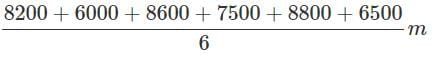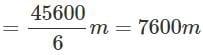Important Questions: Data Handling - Question 15

The heights of six mountains are 8200 m, 6000 m, 8600 m, 7500 m, 8800 m and 6500 m. Based on this information, answer the questions given. Find the median height of the mountains.

Detailed Solution for Important Questions: Data Handling - Question 15

Arranging the heights in ascending order, we have 6000, 6500, 7500, 8200, 8600, 8800.
∴  Median height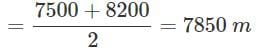Important Questions: Data Handling - Question 16

The heights of six mountains are 8200 m, 6000 m, 8600 m, 7500 m, 8800 m and 6500 m. Based on this information, answer the questions given.
Q. What is the mode of the heights?

Important Questions: Data Handling - Question 17

The heights of six mountains are 8200 m, 6000 m, 8600 m, 7500 m, 8800 m and 6500 m. Based on this information, answer the questions given.
Q. Which of the following statements is true?

Detailed Solution for Important Questions: Data Handling - Question 17

Arranging in ascending order=6000,6500,7500,8200,8600,8800
Median=7500+8200/2=15700/2=7850
Mean=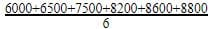= 7600
So, median height is greater than mean height.

Important Questions: Data Handling - Question 18

The heights of six mountains are 8200 m, 6000 m, 8600 m, 7500 m, 8800 m and 6500 m. Based on this information, answer the questions given. Rakesh and Sanjay planned to go trekking on any of these mountains. They wrote the heights on chits of paper, shuffled them and picked one.
Q. What is the probability that the height picked is the maximum?

Important Questions: Data Handling - Question 19

The ages (in years) of some teachers of a school are given in the box. 26, 32,38, 41,26, 31, 35,33,26,37 Based on this information, answer the following questions.
Q. What is the range of the ages of the teachers?

Important Questions: Data Handling - Question 20

The ages (in years) of some teachers of a school are given in the box. 26, 32,38, 41,26, 31, 35,33,26,37 Based on this information, answer the following questions.
Q. What is the mean age of the teachers?

Detailed Solution for Important Questions: Data Handling - Question 20

Mean =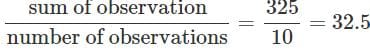years.

## Online MCQ Tests for Class 7

178 tests
 Use Code STAYHOME200 and get INR 200 additional OFF Use Coupon Code
Information about Important Questions: Data Handling Page
In this test you can find the Exam questions for Important Questions: Data Handling solved & explained in the simplest way possible. Besides giving Questions and answers for Important Questions: Data Handling, EduRev gives you an ample number of Online tests for practice

178 tests